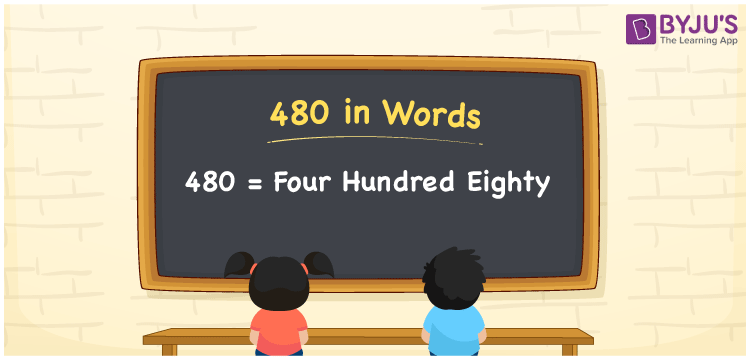# 480 in Words

We can write 480 in words as Four hundred eighty. Suppose the sum of four distinct angles of a geometric shape is 480°, we can express it as “The sum of distinct angles of a geometric shape is Four hundred eighty degrees”. We use the word form of numbers to spell or express a certain quantity or measures. Let’s learn how to write the number 480 in words here in this article.

 480 in words Four hundred eighty Four hundred eighty in Numbers 480

## 480 in English Words

We generally use the English alphabet to write numbers in words. Therefore, we read 480 in English words as “Four hundred eighty”.## How to Write 480 in Words?

The number 480 has three digits. Hence, we need to make a place value chart with three columns as shown below.

 Hundreds Tens Ones 4 8 0

Here, ones = 0, tens = 8, hundreds = 4

The above digits can be expanded as follows.

4 × Hundred + 8 × Ten + 0 × One

= 4 × 100 + 8 × 10

= 400 + 80

= Four hundred + Eighty

= Four hundred eighty

Therefore, 480 in words = Four hundred eighty

We know that 480 is a natural number that precedes 481 and succeeds 479.

480 in words – Four hundred eighty

Is 480 an even number? – Yes

Is 480 an odd number? – No

Is 480 a prime number? – No

Is 480 a composite number? – Yes

Is 480 a perfect square number? – No

Is 480 a perfect cube number? – No

## Frequently Asked Questions on 480 in Words

Q1

### How do you write 480 in words?

We can write the number 480 in English words as Four hundred eighty.
Q2

### Write the value of 18 + 480 in words.

18 + 480 = 498 Thus, the value of 18 + 480, i.e. 498 in words Four hundred ninety-eight.
Q3

### How to write Rs. 480 in words on a cheque?

On a cheque, we can write Rs. 480 in words as “Four hundred eighty rupees only”.